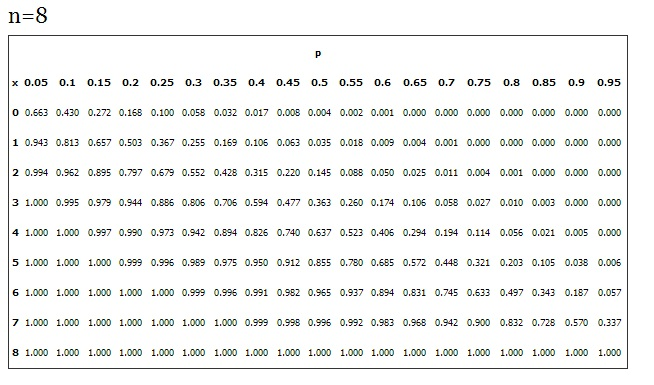# Probability Distribution Table: What is it?

Share on

Statistics Definitions > Probability Distribution Table

## What is a Probability Distribution Table?

A probability distribution table links every outcome of a statistical experiment with the probability of the event occurring. The outcome of an experiment is listed as a random variable, usually written as a capital letter (for example, X or Y). For example, if you were to toss a coin three times, the possible outcomes are:

TTT, TTH, THT, HTT, THH, HTH, HHT, HHH

You have a 1 out of 8 chance of getting no heads at all if you throw TTT. The probability is 1/8 or 0.125, a 3/8 or 0.375 chance of throwing one head with TTH, THT, and HTT, a 3/8 or 0.375 chance of throwing two heads with either THH, HTH, or HHT, and a 1/8 or .125 chance of getting three heads.

The following table lists the random variable (the number of heads) along with the probability of you getting either 0, 1, 2, or 3 heads.

 Number of heads (X) Probability P(X) 0 0.125 1 0.375 2 0.375 3 0.125

Probabilities are written as numbers between 0 and 1; 0 means there is no chance at all, while 1 means that the event is certain. The sum of all probabilities for an experiment is always 1, because if you conduct and experiment, something is bound to happen! For the coin toss example, 0.125 + 0.375 + 0.375 + 0.125 = 1.

Need help with a homework question? Check out our tutoring page!

## More complex probability distribution tables

Of course, not all probability tables are quite as simple as this one. For example, the binomial distribution table lists common probabilities for values of n (the number of trials in an experiment).The more times an experiment is run, the more possible outcomes there are. the above table shows probabilities for n = 8, and as you can see — the table is quite large. However, what this means is that you, as an experimenter, don’t have to go through the trouble of writing out all of the possible outcomes (like the coin toss outcomes of TTT, TTH, THT, HTT, THH, HTH, HHT, HHH) for each experiment you run. Instead, you can refer to a probability distribution table that fits your experiment.

Check out our YouTube channel for more stats help and tips!

## References

Everitt, B. S.; Skrondal, A. (2010), The Cambridge Dictionary of Statistics, Cambridge University Press.
Vogt, W.P. (2005). Dictionary of Statistics & Methodology: A Nontechnical Guide for the Social Sciences. SAGE.
Lindstrom, D. (2010). Schaum’s Easy Outline of Statistics, Second Edition (Schaum’s Easy Outlines) 2nd Edition. McGraw-Hill Education

CITE THIS AS:
Stephanie Glen. "Probability Distribution Table: What is it?" From StatisticsHowTo.com: Elementary Statistics for the rest of us! https://www.statisticshowto.com/what-is-a-probability-distribution-table/
---------------------------------------------------------------------------Need help with a homework or test question? With Chegg Study, you can get step-by-step solutions to your questions from an expert in the field. Your first 30 minutes with a Chegg tutor is free!